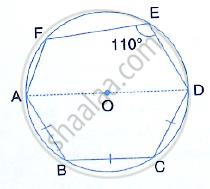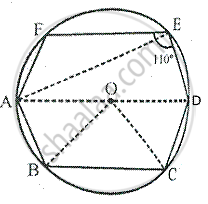Share

Books Shortlist

# In the Following Figure, Ad is the Diameter of the Circle with Centre O. Chords Ab, Bc and Cd Are Equal. If ∠Def = 110°, Calculate: (I) ∠Aef, (Ii) ∠Fab - ICSE Class 10 - Mathematics

ConceptArc and Chord Properties - Angle in a Semi-circle is a Right Angle

#### Question

In the following figure, AD is the diameter of the circle with centre O. chords AB, BC and CD are equal. If ∠DEF = 110°, Calculate:
(i) ∠AEF, (ii) ∠FAB#### SolutionJoin AE, OB and OC
(i) ∵ AOD is the diameter,
∴ ∠AED = 90°                         [Angle in a semi-circle]
But ∠DEF = 110°         [given]
∴ ∠AEF = ∠DEF - ∠AED
= 110° - 90° = 20°

(ii) ∵ Chord AB = Chord BC = Chord CD          [given]

∴∠AOB = ∠BOC = ∠COD         (Equal chords subtends equal angles at the centre)

But ∠AOB + ∠BOC + ∠COD = 180°         [ AOD is a straight line ]
∠AOB = ∠BOC = ∠COD = 60°
In ∠OAB, OA = OB

∴ ∠OAB = ∠OBA                        [radii of the same circle]

But ∠OAB + ∠OBA = 180° - AOB
= 180° - 60°
= 120°
∴ ∠OAB = ∠OBA = 60°